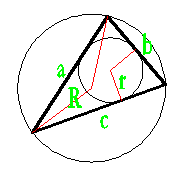## Inscribed circle of a Triangle calculator

 Side a: Side b: Side c: Inscribed Circle Radius : Inscribed Circle Area : Inscribed Circle Perimeter :

every triangle has a unique inscribed circle (incircle) that is interior to the triangle and tangent to all three sides.

1. Radius (r) = √s(s-a)(s-b)(s-c) / s
2. s = (a + b + c) / 2Thinkcalculator.com provides you helpful and handy calculator resources.